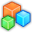# Microsoft Small BasicProgram Listing: RDH625
Timer.Interval=10
Timer.Tick = ProcessCycle
xinc=5
yinc=5
Sub ProcessCycle
batx = Mouse.MouseX-GraphicsWindow.Left
Shapes.Move(bat,batx,400)
ballx = ballx+xinc
bally = bally+yinc
Shapes.Move(ball,ballx,bally)

'check collision!
If(bally > 395) Then
If(ballx > batx And ballx < batx+60) Then
yinc = -yinc
EndIf
EndIf

If(ballx > GraphicsWindow.Width) Then
xinc = -xinc
EndIf
If(ballx < 0) Then
xinc = -xinc
EndIf
If(bally < 0) Then
yinc = -yinc
EndIf
EndSub